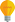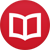# Integral of $\log(x)$ (or $\ln(x)$)

by Jakub MarianTip: See my list of theMost Common Mistakes in English. It will teach you how to avoid mis­takes with com­mas, pre­pos­i­tions, ir­reg­u­lar verbs, and much more.

The natural (base $e$) logarithm is usually denoted $\log(x)$ in mathematics, but for the sake of students of other disciplines, we will denote it $\ln(x)$ in this article.

How to go about the integration? The task is actually very simple with the help of integration by parts, but it requires a little trick. As you can see, there is only one function in

$$∫ \ln(x)\,dx\,,$$

but integration by parts requires two. The trick is to write $\ln(x)$ as $1⋅\ln(x)$ and then apply integration by parts by integrating the $1$ and differentiating the logarithm:

$$∫ \ln(x)\,dx = ∫ \overbrace{1\vphantom{\ln(x)}}^{u’}\overbrace{\ln(x)}^v\,dx = \overbrace{x\vphantom{\ln(x)}}^u\overbrace{\ln(x)}^v - ∫ \overbrace{x\vphantom{\frac1x}}^u\overbrace{\frac1x}^{v’}\,dx = x\ln(x)-x+c\,.$$

Finally, if you found this article because you are wondering what the logarithm of $\log_{10}(x)$ is, then you can use the equality $\log_{10}(x) = \ln(x)/\ln(10)$, so

$$∫ \log_{10}(x)\,dx = ∫ \frac{\ln(x)}{\ln(10)}\,dx = \frac{1}{\ln(10)} ∫ \ln(x)\,dx\,.$$

To get the integral, simply multiply the result above by $\frac{1}{\ln(10)}$.

By the way, I have written several educational ebooks. If you get a copy, you can learn new things and support this website at the same time—why don’t you check them out?